# Notes on designing a pitch shift

Submitted by mic on Fri, 03/30/2018 - 10:41

admin: First posted on 2016 07 12

Guest post by Anton Kamenov

The simplest way to change the pitch of a signal is to simply stretch it or compress it in time. This type of pitch shifting has a disadvantage though, as it also introduces a change in the timing of the signal – it speeds it up or slows it down. It can still be useful, if the signal is short or if its tempo is unimportant. It can be used, for example, for changing the tone of the drum samples used by drum machines or the notes of the wave samples used by MIDI wave mappers.

For example, the operation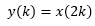will take a signal x(k) of length T and shrink it into a signal y(k) of length T / 2. The cycle of every frequency in the signal will become twice as small, making every frequency twice as large, and shifting the signal one octave higher. The signal x(k) will be re-sampled at half of the original sampling rate and, theoretically, can be re-sampled at any rate to achieve any change in pitch. If x(k) was a digitally recorded song, then y(k) would be the same song an octave higher, but also with a tempo that is twice as fast.

## Better pitch shifting

The discrete Fourier transform (DFT) allows us to decompose a signal into simple wave components with known frequency, magnitude, and phase. We can modify these components by changing their frequencies, and we can use the inverse discrete Fourier transform on these new components to construct a new signal.

The DFT has two disadvantages. First, we cannot use the transform on a long section of the signal, as the composition of the signal may change – a recording of a musical instrument could play different notes or the same notes but with different amplitude at different points of time. The magnitude and phase amounts that we get from the transform are informative for a short period of time, but are meaningless for a longer period of time. The inverse transform will construct a pitch shifted signal that does not vary in time. If we want to use the DFT, we have to do so on small sections of the signal.

Second, the transform cannot produce the magnitude and phase of all frequencies in the signal. It can only do so for a discrete set of selected frequencies with a number equal to the length of the transform. This also makes the transform is imperfect. It produces magnitudes and phase amounts even for simple waves with frequencies that are not exactly the set of frequencies in the discrete transform. For example, a simple wave with frequency 40 Hz may show up with some magnitude and phase at 50 Hz, if we use a DFT at 0 Hz, 50 Hz, 100 Hz, etc. We have to somehow distinguish between the transform frequencies and the actual signal frequencies.

## Handling the lack of precision with frequencies

Let us construct a simple example. Take the complex signal that consists of the three simple waves (simple sine waves) with frequencies 40 Hz, 222 Hz, and 465 Hz, with respective delay of 8, 3, and 2 samples, and with peak amplitudes of 0.3, 1.2, and 0.8 respectively, and suppose that this signal is sampled at 1000 Hz. The three simple waves could be 0.3 sin(2π 40 (k – 8) / 1000), 1.2 sin(2π 222 (k – 3) / 1000), and 0.8 sin(2π 465 (k – 2) / 1000). Since the wave with frequency 40 Hz has a cycle of 1000 / 40 = 25 samples, the delay in 8 samples in the frequency 40 Hz can be translated to a delay of 8 / (1000 / 40) = 0.32 portions of the wave's cycle, or 2π 0.32 = 2.01 radians. Similarly, the phase of the wave at 222 Hz is 4.19 radians, and the phase of the wave at 465 Hz is 5.84 radians.

Suppose that we have a digital representation of the complex signal above and that we do not know anything about the simple waves of the signal (i.e., we do not know their exact frequencies or phase). We begin by taking a short, say, 40 point DFT over the first 40 samples of the signal. Since we are working with the sampling frequency 1000 Hz, a 40-point transform will return magnitude and phase information for 40 components evenly spaced between 0 Hz and 1000 Hz, namely the components 0 Hz, 25 Hz, 50 Hz, …, 975 Hz. For example, at the 25 Hz and 50 Hz components we will get magnitudes of 2.41 and 5.05 respectively. The corresponding phases would be 1.89 radians and -1.53 radians. We could guess that the actual simple frequency existing in the signal is somewhere between 25 Hz and 50 Hz, but at this point we do not have enough information to know what it is precisely.

We continue by taking a 40-point DFT of the next 40 samples in the signal. We get magnitude and phase of 3.22 and -2.45 at 25 Hz and 4.59 and 1.30 at 50 Hz. The phase at 50 Hz started at -1.53 and became -1.30. We would have expected that a wave at 50 Hz, which has a cycle of 1000 / 50 = 20 samples and starts at -1.53 radians, would remain at -1.53 radians after 40 samples, adjusted for two full cycles of 4π. Instead, we get -1.30 radians. We can use this difference in phase to estimate the actual frequency present in the signal.

Consider two simple waves with different frequencies f1 and f2 over the sampling frequency fs and with zero initial phase. These waves are sin(2π f1 k / fs) and sin(2π f2 k / fs). After n samples, these waves would be at phase -2π f1 n / fs and -2π f2 k / fs respectively. If we denote the difference in phase with Δτ, then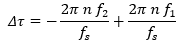and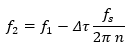Thus, we can compute that the frequency of the wave, which shows up at 50 Hz, is actually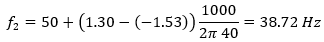This is quite close to the frequency of the actual wave, which, in this example, we know is 40 Hz. It is not exact, but using discrete data never is.

We can estimate the frequencies at all other DFT components, by taking the difference between the phase that we expect for each component at the beginning of the second DFT and the phase that we actually compute with the second DFT. We get the frequency 211 Hz, an estimate for 222 Hz, at the 250 Hz DFT component. We would get 460 Hz at the 475 Hz component, an estimate for 465 Hz.

We must be careful to always adjust the expected phase at the beginning of the second DFT for the number of cycles that the simple wave at that component may have gone through during the length of the DFT. At the 250 Hz component of the first DFT, for example, we would compute the phase -0.41 radians. A wave of 250 Hz, given the sampling frequency of 1000 Hz, would go through 10 cycles until the second DFT begins. Thus, its expected phase at the beginning of the second DFT would be -63.24 radians, but we can adjust this phase for 10 * 2π radians to get, again, -0.41. This is the expected phase at the beginning of the second DFT, which we can compare to the phase computed by the second DFT. In short, if we want to get comparable phase differences between the first and the second DFT, we must adjust the phase that we expect at the beginning of the second DFT by 2π until the difference between this expected phase and the actual second DFT phase is between –π and π.

We will, of course, get estimated frequencies for all components of the DFT, including components that are not even close to the actual frequencies. These are important and should be preserved, if we are to recreate the signal properly with the inverse DFT.

## Working with pieces of the signal

Some of the estimated actual frequencies of the DFT components will seem strange. For example, at the 75 Hz DFT component, we would compute an estimated frequency of 119 Hz (with a relatively low magnitude, of course). It is interesting that 119 Hz shows up in this component. We would have expected it at the 100 Hz component or the 125 Hz component. This could be normal. Any simple frequency would show up with some magnitude, even if really small, in all DFT components.

We can continue taking the DFT at every subsequent segment of the signal – at every 40 samples, and we can pitch shift the resulting frequencies. If, for example, we wanted to raise the pitch by one semitone, we can multiply all recorded frequencies by 21/12 – an equally tempered octave consists of twelve equally spaced semitones and one octave represents a doubling of frequencies.

Once we have completed the pitch shifting, we can begin to recreate the pitch shifted signal. We compute the change in phase for the new true frequencies, attach them to the appropriate component using their original magnitude, and apply the inverse DFT. Here, however, we run into a problem. We would be recreating the signal 40 samples at a time. At the border of each 40 sample interval, we run the risk of having discontinuities.

A discontinuity, such as the one in the figure below, would likely result in an audible click in the signal. The best way to avoid discontinuities is to cross-fade, which means mixing the end of the first portion with the beginning of the second portion, by slowly decreasing the amplitude of the first portion and increasing the amplitude of the second portion. A simple straight cross fading is shown on the next figure, where the dashed lines represent the gains applied to each of the two portions. To do so, however, we need overlapping portions.x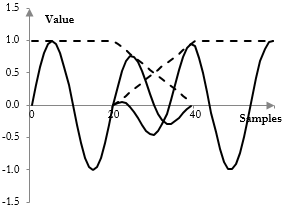To implement a working pitch shift, we need to apply the DFT to overlapping portions of the signal. If the DFT itself uses 40 points, then the second portion could start not at sample 40, but at sample 20, giving a half segment overlap and allowing for cross fading.

Overlapping also helps with the estimating of actual frequencies. In the equation from above,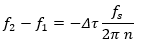since we keep –π  Δτ  π, then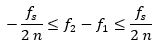If the size of the DFT is N, then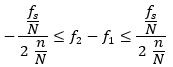Each DFT component then is "responsible" for fs / N frequencies. When n / N = 1 / 2 – the DFTs overlap by a half – then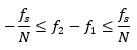and we can ensure that the estimated frequency does not jump over into another DFT component. For example, if we overlap the example DFTs above by 20 points – half of 40 points – instead of estimating that the actual frequency at the 75 Hz component is 119 Hz, we would get 88 Hz, which is closer to the component. Instead of 38.72 Hz at the 50 Hz component, we would get 39.41 Hz. This is a better estimate of the actual frequency in the complex signal, which is 40 Hz.

In summary, a good pitch shift can be performed by: 1) taking the DFT of consecutive segments of the signal that overlap by at least a half; 2) noting magnitude and the difference between the expected phase and the actual phase computed at the beginning of each DFT (except for the first one) and estimating the actual frequency of the segment for each DFT component; 3) adjusting the estimated frequencies for the change in pitch; 4) computing new phase amounts and the inverse DFT; and 5) cross fading the signal from the inverse DFT segments for the overlap.

The same algorithm can be applied to time stretching or shrinking a signal if the goal is to change the tempo of the signal without changing its pitch. The only difference would be that rather than adjusting the frequencies of the segments for pitch, we would adjust the timing of the segments before the inverse DFT.

## Add new comment

### Filtered HTML

• Freelinking helps you easily create HTML links. Links take the form of [[indicator:target|Title]]. By default (no indicator): Click to view a local node.
• Web page addresses and e-mail addresses turn into links automatically.
• Lines and paragraphs break automatically.

### Plain text

• No HTML tags allowed.
• Web page addresses and e-mail addresses turn into links automatically.
• Lines and paragraphs break automatically.